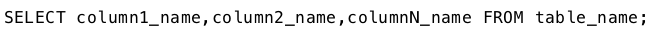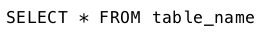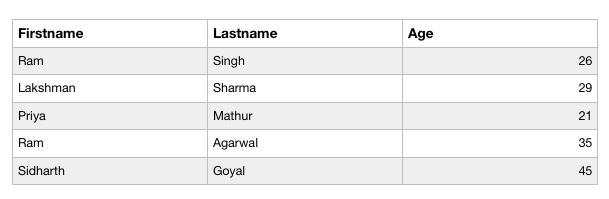# PHP | MySQL Select Query

The SQL SELECT statement is used to select the records from database tables.

Syntax :
The basic syntax of the select clause is –To select all columns from the table, thecharacter is used.Implementation of the Select Query :
Let us consider the following table ‘ Data ‘ with three columns ‘ FirstName ‘, ‘ LastName ‘ and ‘ Age ‘.To select all the data stored in the ‘ Data ‘ table, we will use the code mentioned below.

SELECT Query using Procedural Method :

 ` 0) { ` `        ``echo ``""``; ` `        ``echo ``"
FirstnameLastnameage
"``.\$row[``'Firstname'``].``""``.\$row[``'Lastname'``].``""``.\$row[``'Age'``].``"
"``; ` `        ``echo ``""``; ` `        ``echo ``""``; ` `        ``echo ``""``; ` `        ``echo ``""``; ` `        ``while` `(\$row = mysqli_fetch_array(\$res)) { ` `            ``echo ``""``; ` `            ``echo ``""``; ` `            ``echo ``""``; ` `            ``echo ``""``; ` `            ``echo ``""``; ` `        ``} ` `        ``echo ``""``; ` `        ``mysqli_free_result(\$res); ` `    ``} ` `    ``else` `{ ` `        ``echo ``"No matching records are found."``; ` `    ``} ` `} ` `else` `{ ` `    ``echo ``"ERROR: Could not able to execute \$sql. "` `                                ``.mysqli_error(\$link); ` `} ` `mysqli_close(\$link); ` `?> `

Output :Code Explanation:

1. The “res” variable stores the data that is returned by the function mysql_query().
2. Everytime mysqli_fetch_array() is invoked, it returns the next row from the res() set.
3. The while loop is used to loop through all the rows of the table “data”.

SELECT Query using Object Oriented Method :

 `connect_error); ` `} ` ` `  `\$sql = "SELECT * FROM Data"; ` `if (\$res = \$mysqli->query(\$sql)) { ` `    ``if (\$res->num_rows > 0) { ` `        ``echo "<``table``>"; ` `        ``echo "<``tr``>"; ` `        ``echo "<``th``>Firstname"; ` `        ``echo "<``th``>Lastname"; ` `        ``echo "<``th``>Age"; ` `        ``echo ""; ` `        ``while (\$row = \$res->fetch_array())  ` `        ``{ ` `            ``echo "<``tr``>"; ` `            ``echo "<``td``>".\$row['Firstname'].""; ` `            ``echo "<``td``>".\$row['Lastname'].""; ` `            ``echo "<``td``>".\$row['Age'].""; ` `            ``echo ""; ` `        ``} ` `        ``echo ""; ` `        ``\$res->free(); ` `    ``} ` `    ``else { ` `        ``echo "No matching records are found."; ` `    ``} ` `} ` `else { ` `    ``echo "ERROR: Could not able to execute \$sql. " ` `                                             ``.\$mysqli->error; ` `} ` `\$mysqli->close(); ` `?> `

Output :SELECT Query using PDO Method :

 `setAttribute(PDO::ATTR_ERRMODE,  ` `                        ``PDO::ERRMODE_EXCEPTION); ` `} ` `catch (PDOException \$e) { ` `    ``die("ERROR: Could not connect. ".\$e->getMessage()); ` `} ` `try { ` `    ``\$sql = "SELECT * FROM Data"; ` `    ``\$res = \$pdo->query(\$sql); ` `    ``if (\$res->rowCount() > 0) { ` `        ``echo "<``table``>"; ` `        ``echo "<``tr``>"; ` `        ``echo "<``th``>Firstname"; ` `        ``echo "<``th``>Lastname"; ` `        ``echo "<``th``>Age"; ` `        ``echo ""; ` `        ``while (\$row = \$res->fetch()) { ` `            ``echo "<``tr``>"; ` `            ``echo "<``td``>".\$row['Firstname'].""; ` `            ``echo "<``td``>".\$row['Lastname'].""; ` `            ``echo "<``td``>".\$row['Age'].""; ` `            ``echo ""; ` `        ``} ` `        ``echo ""; ` `        ``unset(\$res); ` `    ``} ` `    ``else { ` `        ``echo "No matching records are found."; ` `    ``} ` `} ` `catch (PDOException \$e) { ` `    ``die("ERROR: Could not able to execute \$sql. " ` `                                ``.\$e->getMessage()); ` `} ` `unset(\$pdo); ` `?> `

Output :Unlock the Power of Placement Preparation!
Feeling lost in OS, DBMS, CN, SQL, and DSA chaos? Our Complete Interview Preparation Course is the ultimate guide to conquer placements. Trusted by over 100,000+ geeks, this course is your roadmap to interview triumph.